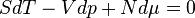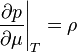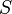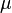# Gibbs-Duhem relation$SdT -Vdp + Nd\mu =0$$\left.\frac{\partial p}{\partial \mu}\right\vert_{T} = \rho$
where$p$ is the pressure,$S$ is the entropy,$V$ is the volume,$\mu$ is the chemical potential and$\rho$ is the number density.